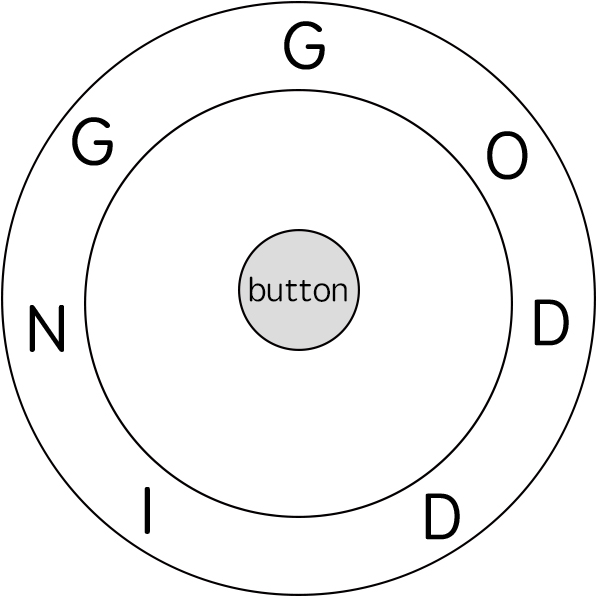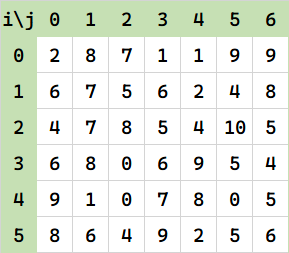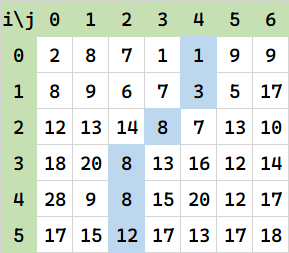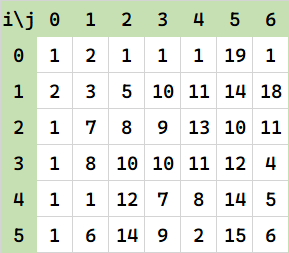### LC 115. 不同的子序列

s = babgbag
t = bag


• 需要满足 $s [i] = t [i]$，即结尾字符相等。否则一定无法匹配。

• 匹配剩余部分：$s [0:i-1]$ 匹配 $t [0:j-1]$

• $s [0:i-1]$ 匹配 $t [0:j]$

f(i,j) = \left\{\begin{align} f(i-1,j) + f(i-1,j-1) &;s[i] = t[i]\\ f(i-1,j) &;\text{else} \end{align}\right.

dp[i][j] = dp[i-1][j];
if (s[i-1]==t[j-1]) dp[i][j]+=dp[i-1][j-1];


### LC 514. 自由之路

1. 您可以将 ring 顺时针或逆时针旋转一个位置，计为 1 步。旋转的最终目的是将字符串 ring 的一个字符与 12:00 方向对齐，并且这个字符必须等于字符 key [i] 。
2. 如果字符 key [i] 已经对齐到 12:00 方向，您需要按下中心按钮进行拼写，这也将算作 1 步。按完之后，您可以开始拼写 key 的下一个字符（下一阶段）, 直至完成所有拼写。输入: ring = "godding", key = "gd"

对于 key 的第二个字符 'd'，我们需要逆时针旋转 ring "godding" 2 步使它变成 "ddinggo"。

因此最终的输出是 4。

• 按下中间算一步
• 左右转一次算一步

## 矩阵坐标型

### 棋盘最小代价路径（Min Cost Path in Chess Board）

/ | \


$f(i,j) = \min\{f(i-1,j-1) ,f(i-1,j), f(i-1,j+1)\} + \text{cost}(i,j)$### 最长增长路径（Longest Increasing Path）

（1）如果允许走下或者右。（2）如果允许走上下左右四个方向